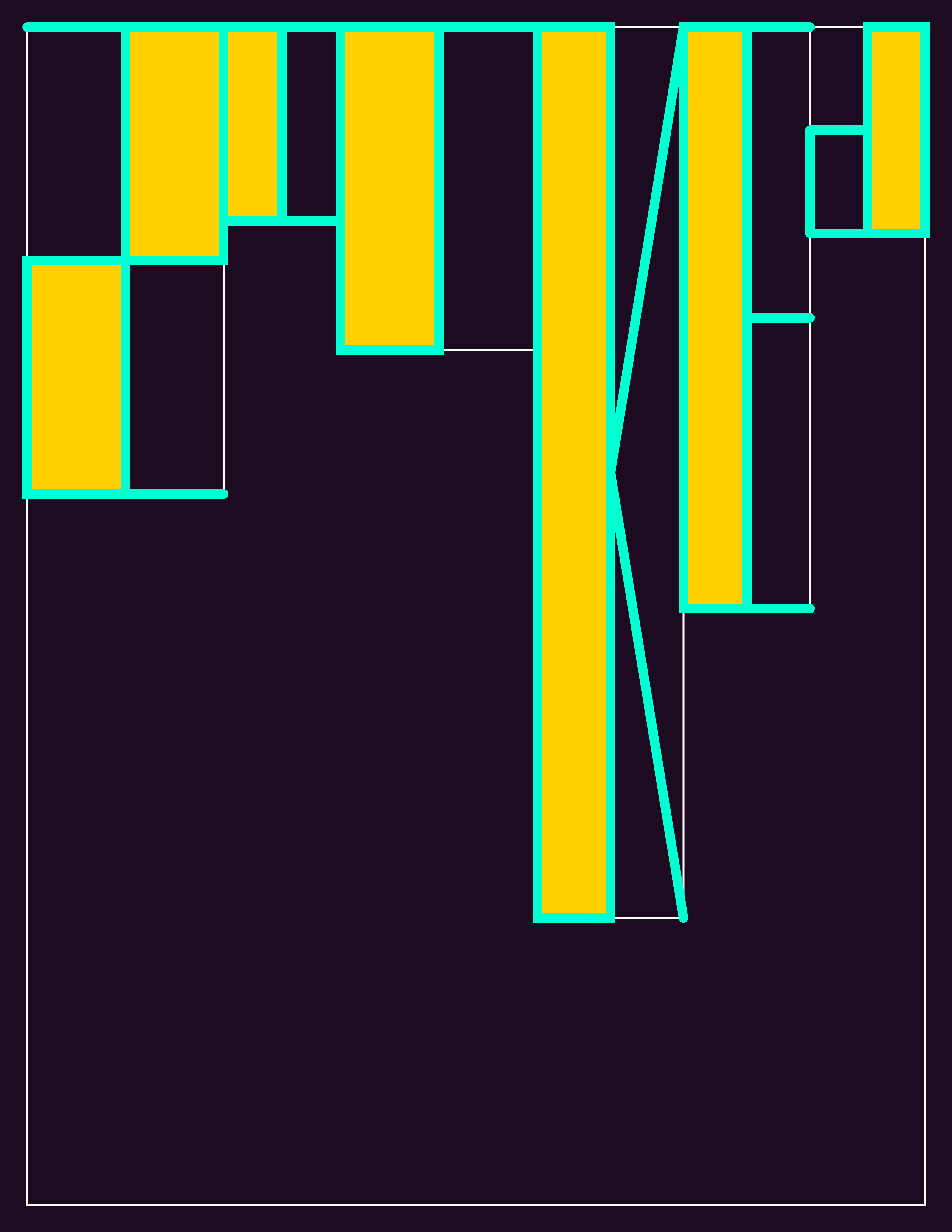# On the Grid

Much of my work for Printing Code, including the generative work, has been very deliberately controlled. Rune challenged us to work outside of our usual style–careful plotters should work more haphazardly, and vice versa. The grid assignment seemed like a good place to start.

One of the examples for class was a randomized column grid, wherein the column widths generate differently every time. For my own program, I chose to randomize both the width and height of the columns. I used these values as size parameters for letters of the font that I designed for the previous assignment.

The bizarre resulting shapes and my minimal hours of sleep as an ITP student made the word “zonked” an appropriate choice.This is an iteration of the program with the gridlines turned on. We were not supposed to print the gridlines on the final poster, but it’s interesting to see how they inform the design.Here is the main code for the sketch. Read the rest of the code on Github.

```1 2 3 4 5 6 7 8 9 10 11 12 13 14 15 16 17 18 19 20 21 22 23 24 25 26 27 28 29 30 31 32 33 34 35 36 37 38 39 40 41 42 43 44 45 46 47 48 49 50 51 52 53 54 55 56 57 58 59 60 61 62 63 64 65 66 67 68 69 70 71 72 73 74 75 76 77 78 79 80 81 82 83 84 85 86 87 88 89 90 91 92 93 94 95 96 97 98 99 100 101 102 103 104 105 106 107 108 109 110 111 112 113 //Kim Ash //Printing Code //gridPoster - randomized grid, letter parameters adjust to column sizes   PGraphics canvas; int canvas_width = 5100; int canvas_height = 6600;   float ratioWidth = 1; float ratioHeight = 1; float ratio = 1;   Letter [] letters;   void setup() { size(510, 660); canvas = createGraphics(canvas_width, canvas_height); calculateResizeRatio(); letters = new Letter;   canvas.beginDraw(); canvas.colorMode(HSB, 360, 100, 100); float h = random(360); canvas.background(h, random(100), random(100));   gridfxn(6, canvas.width/15); canvas.smooth(); canvas.strokeWeight(canvas.width/100); canvas.fill(((h+120) % 360), 100, 100); canvas.stroke(((h+240) % 360), 100, 100); letters.z(); letters.o(); letters.n(); letters.k(); letters.e(); letters.d(); canvas.endDraw();   float resizedWidth = (float) canvas.width * ratio; float resizedHeight = (float) canvas.height * ratio; //displays canvas onscreen image(canvas, (width/2) - (resizedWidth/2), (height/2) - (resizedHeight/2), resizedWidth, resizedHeight);     canvas.save("gridPoster.tiff"); }   /* resizing function */ void calculateResizeRatio() { ratioWidth = (float) width / (float) canvas.width; ratioHeight = (float) height / (float) canvas.height;   if(ratioWidth < ratioHeight) ratio = ratioWidth; else ratio = ratioHeight; }   //void keyPressed() //{ // grid.display(); //}   void gridfxn(int cols, float pageMargin) { Column[] columns; float fullWidth = canvas.width - (2*pageMargin); float[] rands = new float[cols]; float ranSum = 0;   for(int i = 0; i < rands.length; i++) { // generate a number between 0 and 1 rands[i] = random(1); // OPTIONAL! tweak the number to avoid too big a size difference if(rands[i] < 0.4) rands[i] = 0.4; // add to the sum ranSum += rands[i]; }   // now divide each random number with its sum to normalize and multiply by full width for(int i = 0; i < rands.length; i++) { rands[i] = (rands[i] / ranSum) * fullWidth; } columns = new Column[cols]; float xPos = pageMargin;   for(int i = 0; i < cols; i++) { columns[i] = new Column(); columns[i].x = xPos; columns[i].y = pageMargin; columns[i].w = rands[i]; columns[i].h = canvas.height - (2*pageMargin) - (random(1)*canvas.height);   xPos += rands[i];   letters[i] = new Letter(columns[i].x, columns[i].y, (columns[i].w)/2, columns[i].h); }   //bounding box canvas.noFill(); canvas.stroke(0, 0, 100, 0); //change alpha value to see gridlines canvas.strokeWeight(canvas.width/500); canvas.rect(pageMargin, pageMargin, canvas.width - (2*pageMargin), canvas.height - (2*pageMargin));   //grid for(int i = 0; i < cols; i++) { canvas.rect(columns[i].x, columns[i].y, columns[i].w, columns[i].h); } }```